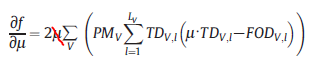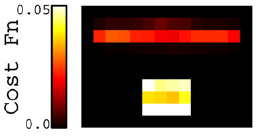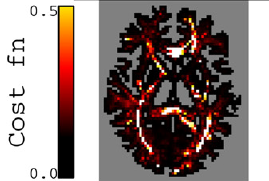# Calculate the cost function in SIFT

Dear MRtrix3 team

I recently read the paper ‘‘SIFT: Spherical-deconvolution informed filtering of tractograms’’.
I have a question about how to calculate the cost function.According to the equationI’m not sure that the u and TD are the values before removing the prospective streamline or after.
Could I understand that using the lobes not traversed by the removal streamline (the equation 4) represent the all lobes (traversed and not traversed) for calculating the cost function (f) ?
One more question about how to calculate _the gradient per unit length for each streamlin_e ,is it using the gradient of a streamline divide the streamline length ?

Hi again,

So that equation (neglecting my math error…) is used to estimate the change in cost function for all lobes, given the change in u only (`src/dwi/tractography/SIFT/sifter.cpp:422`).

For those lobes that are traversed by the streamline, two things happen:

1. The contribution of that lobe to the partial derivative above is removed, by calculating the contribution to that term from that lobe alone and inverting it (`sifter.cpp:425,427`)
2. The difference in the cost function for the prospective removal of that streamline (i.e. both before and after) is calculated precisely, based on both the change in u and the change in TD (`sifter.cpp:426`). We could write this as (using `f()` to represent the cost function):
`df = f (TD_without_track, u_without_track) - f (TD_with_track, u_with_track)`

Yes, the gradient per unit length is literally the gradient divided by the streamline length (`sifter.cpp:442`). Though the ‘length’ used for this scaling (which only affects the order of streamline removal) is actually the sum of (`streamline_length_in_voxel * processing_mask_value_in_voxel`); this should remove any biases in streamline removal order due to different streamlines having different processing mask values along their lengths.

Cheers
Rob

I’m a little understand the exact thought about calculating the f.
One more question about_ the contribution to cost function from each voxel in Fig.4 and Fig.5_.In my view, I think it represents the percentage of the cost function, the sum of contribution from each voxel is 1.But according to color bar in Fig.4(0.05)and Fig.5(0.5)it appears wrong…
So what is the contribution ?

There aren’t any percentages involved here. The simplest figure to understand is the top one. In each individual voxel (that is within one of the two bundles), there is a single FOD lobe. For each, we can solve Equation 3, just for that one lobe (i.e. there are no sums across voxels or lobes):
`f = PM. (u.TD - FOD)^2`
This is the value that determines the colour within each voxel.
The total cost function is then the sum of these values across the entire image.

For the lower image, the only difference is that there may now be multiple lobes in a single voxel. In this case therefore, for each voxel, the sum of the contributions to f from all lobes within that voxel is calculated, and this is the value that determines the colour of that voxel in the image.

We certainly don’t want the sum of all contributions to equal 1: this would remove the information regarding whether one tractogram configuration is a better fit to the data than another. Remember that the cost function f yields a single scalar value across the entire image volume; these figures are simply demonstrating the spatial regions within the image volume that are contributing the greatest amount toward this value.

Dear rsmith
Thank you for your patience to answer my questions！
I recently read the paper “The effects of SIFT on the reproducibility and biological accuracy of the structural connectome”
Just one simple question, how do I understand the meaning of ‘up to a global scaling factor’?
I’m sorry to bother you so many times:sweat:

So there’s a couple of meanings behind using that term (used repeatedly to cover ourselves).

Firstly, we make the argument that the total intra-cellular cross-sectional area between two regions is a reasonable measure for the structural connectivity between them. Ideally we’d have a gamut of measures such as axon count, diameter distributions, myelination etc.; but intra-cellular cross-sectional area is something we can quantify reasonably well.

Ideally we would provide this measure in units of mm^2, ie. an actual physical cross-sectional area. However because the AFD measure itself is only proportional to fibre volume and is not a volume fraction, and is affected by T2 differences, we cannot use the physical size of the image voxels to convert this into a physically-dimensioned measure. Therefore we are stuck with something that is proportional to cross-sectional area; there’s a hidden global scaling factor to convert from our measure (streamline count) to an actual cross-sectional area, but we don’t know what it is.

The other ramification of this effect is that although we can compare the relative connection densities of different pathways in the one reconstruction (provided we perform B1 bias field correction), we cannot trivially compare connection densities directly between subjects. It’s fairly common practise to just use the same number of streamlines in each subject and then compare like with like, but there are a few hidden assumptions when using this approach that many may not be aware of.

Dear rsmith
Thank you very much !
I recently read the paper '‘SIFT2: Enabling dense quantitative assessment of brain white matter connectivity
using streamlines tractography’'
I have a question about why making a combination of whole-brain and targeted seeding. Using SIFT2 ,“the very dense sampling of the plausible pathways emanating from the structure of interest is retained” Is there deeply meaning or other purpose?

When people perform targeted tracking, it’s often because they want to generate a large number of streamlines for a particular pathway; to make sure that the spatial extent of the pathway is well-defined in the reconstruction, and that the pathway is evident from the bulk streamline behaviour rather than counting individual streamlines. You could equivalently generate a whole-brain tractogram, and then extract those streamlines corresponding to your pathway of interest; but the reconstruction density of that pathway may then be not adequate.

For instance: Say you’re interested in the density of a very small pathway. You generate a whole-brain tractogram, apply SIFT (greatly reducing the number of tracks), then select only those in your pathway of interest (also greatly reducing the number of tracks). If the final number of streamlines in your pathway is too small, then you may be susceptible to erroneous conclusions: either due to the spatial extent and termination points of the pathway being ill-defined (because the remaining streamlines are few and far between), or the streamline count being so small that the random nature of the tracking process makes your results highly variable.

This trick is simply a way of combining the quantitative properties attainable using SIFT/SIFT2, with the high targeted reconstruction density of targeted tracking. If you have a particular pathway of interest, you can reconstruct it with a very high density (to make sure that you are not missing any features of that pathway), and combine that data with a whole-brain tractogram (to obtain the quantitative properties). To get a comparable result using exclusively whole-brain tracking, you would either run the risk of your pathway of interest being too sparsely reconstructed, or have to generate a massive number of whole-brain streamlines to ensure that this doesn’t happen.

I’m a little confused about the ‘dynamic seeding’.
‘Each time an FOD lobe is selected at random for potential seeding……… Once an FOD lobe has been selected for streamline seeding, a random position within that voxel is determined’
In my view, firstly, I should select an FOD lobe for potential seeding, but how to select one FOD lobe? Is the FOD in the center of the voxel?
If an FOD lobe has been chosen, then choosing a random position within that voxel? As for the chosen FOD lobe，it just determines the roughly initial seeding direction?

The selection of FOD lobes for seeding is done based on the FOD lobes within your seed mask image. That mask will contain some number of FOD lobes. A lobe is selected entirely at random, and a decision is then made as to whether or not it is appropriate to seed a streamline from that lobe (if it is not, another lobe is selected at random, and the process repeats). So you as a user do not need to select an FOD lobe for seeding: this is done intrinsically by the dynamic seeding algorithm.

The position within the voxel from which to propagate the streamline is also determined automatically by the seeding algorithm. It’s preferable to not seed all streamlines from the very centre of a voxel, as this would introduce a bias into the coverage of streamlines emanating from that voxel.

The FOD lobe used to determine the streamline seed position will also provide a fibre direction. However, the tracking algorithm must then initialize correctly based on this seed direction: precisely what occurs here will depend on whether a probabilistic or deterministic tracking algorithm is used.